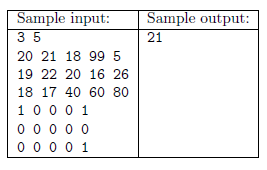Mathematical and Physical Journal
for High Schools
Issued by the MATFUND Foundation
 Already signed up? New to KöMaL?

#Problem S. 101. (October 2015)

S. 101. We are given the map of a mountain range as a grid of $\displaystyle N\times M$ numbers containing the elevation data for each coordinate (every elevation is at most $\displaystyle 1 \,000\,000\,000$. We are also given some nice locations in the mountains. We would like to determine at least how difficult a trip is which starts from some nice location and visits every nice location. In other words, we aim to determine the smallest number $\displaystyle C$ with the following property: it is possible to start from any nice location and arrive at any other nice location by always proceeding from a square to one of its 4 neighbors with elevation difference at most $\displaystyle C$.

First your program should read the values of $\displaystyle N$ and $\displaystyle M$ ($\displaystyle 1\le N, M\le 800$, being the number of rows and columns of the map, respectively) from the first line of the standard input. Then read $\displaystyle M$ integers, representing elevation data, from each of the following $\displaystyle N$ lines. Next read again $\displaystyle M$ integers from each of the following $\displaystyle N$ lines: every integer is either 0 or 1 depending on whether the current location is nice or not, respectively. Your program should write the smallest possible appropriate $\displaystyle C$ in the first and only line of the standard output.Scoring and bounds. You can get 1 point for a brief and proper documentation clearly describing your solution. Nine further points can be obtained provided that your program solves any valid input within 1 second of running time.

The source code of your program without the .exe or any other auxiliary files generated by the compiler but with a short documentation$\displaystyle -$also describing which developer environment to use for compiling the source$\displaystyle -$should be submitted in a compressed file s101.zip.

(10 pont)

Deadline expired on November 10, 2015.

### Statistics:

 22 students sent a solution. 10 points: Bálint Martin, Csenger Géza, Fuisz Gábor, Gáspár Attila, Gergely Patrik, Janzer Orsolya Lili, Németh 123 Balázs, Noszály Áron, Zalavári Márton, Zarándy Álmos. 9 points: Alexy Marcell. 8 points: 1 student. 7 points: 1 student. 6 points: 1 student. 5 points: 3 students. 3 points: 2 students. 1 point: 1 student. 0 point: 2 students.

Problems in Information Technology of KöMaL, October 2015